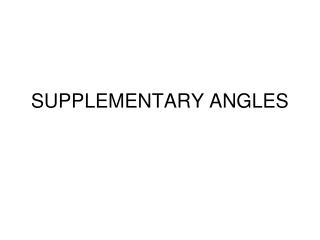DownloadDownload PresentationSUPPLEMENTARY ANGLES

# SUPPLEMENTARY ANGLES

Télécharger la présentation## SUPPLEMENTARY ANGLES

- - - - - - - - - - - - - - - - - - - - - - - - - - - E N D - - - - - - - - - - - - - - - - - - - - - - - - - - -
##### Presentation Transcript

1. SUPPLEMENTARY ANGLES

2. 2-angles that add up to 180 degrees.

3. COMPLEMENTARY ANGLES

4. 2-angles that add up to 90 degrees

5. Vertical Angles

6. Vertical Angles are Congruent to each other • <1 =<3 • <2=<4 • <1+<2=180 degrees • <2+<3=180 degrees • <3+<4=180 degrees • <4+<1=180 degrees

7. PARALLEL LINES CUT BY A TRANSVERSAL

8. SUM OF THE INTERIOR ANGLES OF A TRIANGLE

9. 180 DEGREES

10. EQUILATERAL TRIANGLE

11. Triangle with equal angles and equal sides

12. ISOSCELESTRIANGLE

13. TRIANGLE WITH 2 SIDES = AND 2 BASE ANGLES =

14. ISOSCELES RIGHT TRIANGLE

15. RIGHT TRIANGLE WITH BC=CA and < A = <B

16. EXTERIOR ANGLE THEOREM

17. LARGEST ANGLE OF A TRIANGLE

18. ACROSS FROM THE LONGEST SIDE

19. SMALLEST ANGLE OF A TRIANGLE

20. ACROSS FROM THE SHORTEST SIDE

21. LONGEST SIDE OF A TRIANGLE

22. ACROSS FROM THE LARGEST ANGLE

23. SMALLEST SIDE OF A TRIANGLE

24. ACROSS FROM THE SMALLEST ANGLE

25. TRIANGLE INEQUALITY THEOREM

26. PROPORTIONS IN THE RIGHT TRIANGLE

27. (Upside down T!!!!) (Big Angle Small Angle!!!!!)

28. CONCURRENCY OF THE THE ANGLE BISECTORS

29. INCENTER

30. CONCURRENCY OF THE PERPENDICULAR BISECTORS

31. CIRCUMCENTER

32. CONCURRENCY OF THE MEDIANS

33. CENTROID MEDIANS ARE IN A RATIO OF 2:1

34. CONCURRENCY OF THE ALTITUDES

35. ORTHOCENTER

36. Properties of a Parallelogram

37. Parallelogram • Opposite sides are congruent. • Opposite sides are parallel. • Opposite angles are congruent. • Diagonals bisect each other. • Consecutive (adjacent) angles are supplementary (+ 180 degrees). • Sum of the interior angles is 360 degrees.

38. Properties of a Rectangle

39. Rectangle • All properties of a parallelogram. • All angles are 90 degrees. • Diagonals are congruent.

40. Properties of a Rhombus

41. Rhombus • All properties of a parallelogram. • Diagonals are perpendicular (form right angles). • Diagonals bisect the angles.

42. Properties of a Square

43. Square • All properties of a parallelogram. • All properties of a rectangle. • All properties of a rhombus.

44. Properties of an Isosceles Trapezoid

45. Isosceles Trapezoid • Diagonals are congruent. • Opposite angles are supplementary + 180 degrees. • Legs are congruent

46. Median of a Trapezoid